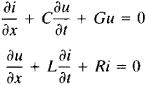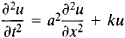# Telegrapher's Equation

(redirected from Telegrapher's equations)
Also found in: Wikipedia.

## telegrapher's equation

[tə′leg·rə·fərz i‚kwā·zhən]
(mathematics)
The partial differential equation (∂2ƒ/∂ x 2) = a 2(∂2ƒ/∂ y 2) + b (∂ƒ/∂ y) + c ƒ, where a, b, and c are constants; appears in the study of atomic phenomena.

## Telegrapher’s Equation

(or equation of telegraphy), in mathematics, a partial differential equation that, under certain simplifying assumptions, describes the propagation of a current along a conductor.

The current i and voltage u satisfy the following system of equations:Here, x is a coordinate reckoned along the conductor, t is time, and C, G, L, and R are the capacitance, conductance, inductance, and resistance, respectively, of the conductor per unit length. If LC ≠ 0, a suitable change of variables leads to the equationwhich is one form of the telegrapher’s equation.

Boundary value problems for the telegrapher’s equation are solved by methods developed for the equation of vibration of a string (seeWAVE EQUATION). The telegrapher’s equation reduces to this equation when k = 0. When k ≠ 0, a dispersion phenomenon exists in the process described by the telegrapher’s equation (see, for example, DISPERSION OF SOUND). Operational calculus and special functions are commonly used to solve the telegrapher’s equation.

The telegrapher’s equation was studied by Lord Kelvin in 1855 for the case L = 0. G. Kirchhoff dealt with the general case in 1857. Others who have investigated the equation include O. Heaviside in 1876 and H. Poincaré in 1897. The term “telegrapher’s equation” was suggested by Poincaré (Téquation destélégraphistes).

References in periodicals archive ?
This theory presents a rigorous derivation of Maxwell's equations for non-uniform transmission lines into a set of coupled equations which resembles the telegrapher's equations. The per-unit-length (p.u.l.) parameters, which are position and frequency-dependent, are calculated through a rather complex iterative procedure.
In the hypothesis that the field structure is Transverse ElectroMagnetic (TEM) or quasi-TEM the voltage distribution across the coil can be calculated by using the MTL theory and the telegrapher's equations.
Transmission lines are known to satisfy the telegrapher's equations, which (in the time domain) are given by
This analysis is done using transmission line theory that involves solving the telegrapher's equations in time domain along with the nonlinear loads and drivers.
The well known, FDTD technique  is used to solve the lossless version of telegrapher's Equations (1) and (2).
The response of these towers for direct lightning waves can be modeled by the telegrapher's equations of transmission lines (TL):
Crosstalk analysis has been studied for parallel MTLs using network functions based on the telegrapher's equations under the assumption of transverse electromagnetic (TEM) mode propagation or at least quasi-TEM mode propagation [1,2].
This coupling of external fields to transmission lines can be expressed in the so-called mod[I.sub.fi]ed telegrapher's equations. The differential equations regarding line voltage and current, which were derived from Maxwell's equations, contain forcing terms in external EM-field components [4-6].
Furthermore, the results obtained by the proposed approach are compared with those obtained by the conventional telegrapher's equations.
One example is the so-called telegrapher's equation, an equation described by a 2x2 hyperbolic balance law and normally used to model transmission lines.
If [Z.sub.e] = [R.sub.e] = 0, the telegrapher's equation reduces to the linear wave equation.
We have introduced a feedback stabilization result for general network topologies and applied it to the telegrapher's equation and the LWR traffic flow model.
Site: Follow: Share:
Open / Close